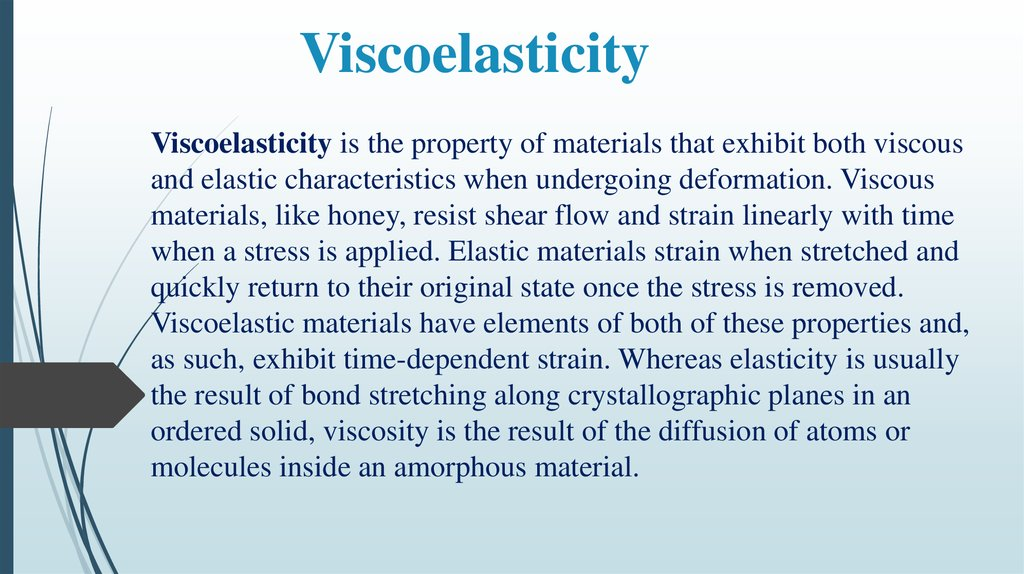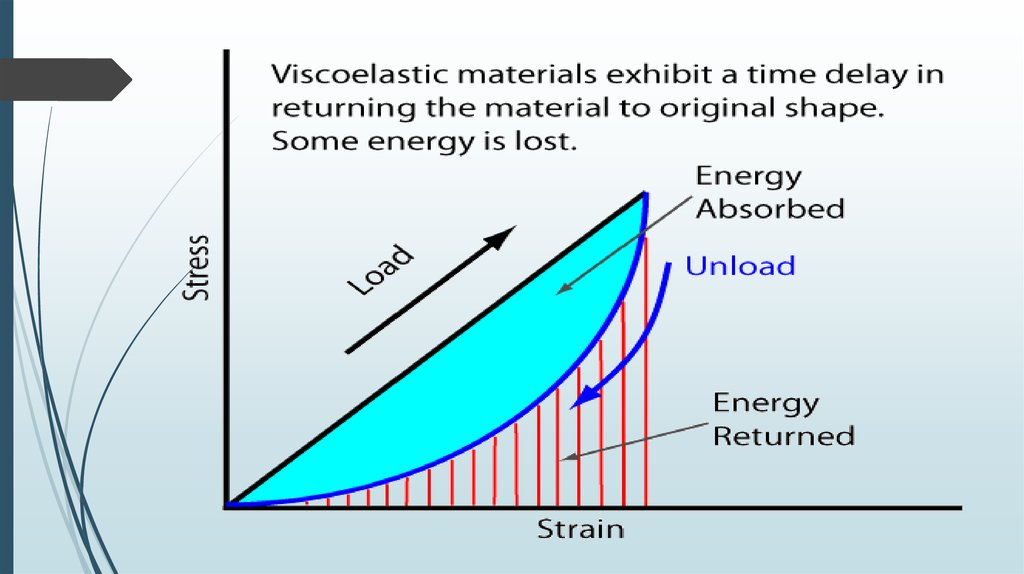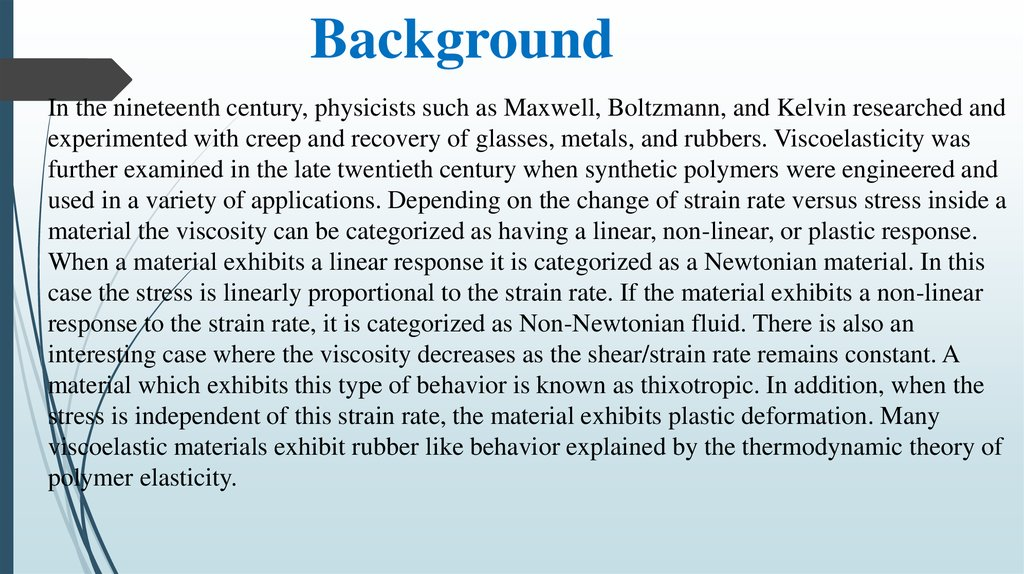# Viscoelasticity

## 1. Viscoelasticity

Viscoelasticity is the property of materials that exhibit both viscous
and elastic characteristics when undergoing deformation. Viscous
materials, like honey, resist shear flow and strain linearly with time
when a stress is applied. Elastic materials strain when stretched and
quickly return to their original state once the stress is removed.
Viscoelastic materials have elements of both of these properties and,
as such, exhibit time-dependent strain. Whereas elasticity is usually
the result of bond stretching along crystallographic planes in an
ordered solid, viscosity is the result of the diffusion of atoms or
molecules inside an amorphous material.

## 3.

Background
In the nineteenth century, physicists such as Maxwell, Boltzmann, and Kelvin researched and
experimented with creep and recovery of glasses, metals, and rubbers. Viscoelasticity was
further examined in the late twentieth century when synthetic polymers were engineered and
used in a variety of applications. Depending on the change of strain rate versus stress inside a
material the viscosity can be categorized as having a linear, non-linear, or plastic response.
When a material exhibits a linear response it is categorized as a Newtonian material. In this
case the stress is linearly proportional to the strain rate. If the material exhibits a non-linear
response to the strain rate, it is categorized as Non-Newtonian fluid. There is also an
interesting case where the viscosity decreases as the shear/strain rate remains constant. A
material which exhibits this type of behavior is known as thixotropic. In addition, when the
stress is independent of this strain rate, the material exhibits plastic deformation. Many
viscoelastic materials exhibit rubber like behavior explained by the thermodynamic theory of
polymer elasticity.

## 5. Types

Linear viscoelasticity is when the function is separable in both creep response
and load. All linear viscoelastic models can be represented by a Volterra equation
connecting stress and strain:
Linear viscoelasticity is usually applicable only for small deformations.
Nonlinear viscoelasticity is when the function is not separable. It usually happens
when the deformations are large or if the material changes its properties under
deformations.
An anelastic material is a special case of a viscoelastic material: an anelastic material
will fully recover to its original state on the removal of load.

## 6. Dynamic modulus

Viscoelasticity is studied using dynamic mechanical analysis,
applying a small oscillatory stress and measuring the resulting
strain.
Purely elastic materials have stress and strain in phase, so that the
response of one caused by the other is immediate.
In purely viscous materials, strain lags stress by a 90 degree phase
lag.
Viscoelastic materials exhibit behavior somewhere in the middle of
these two types of material, exhibiting some lag in strain.

## 7. Constitutive models of linear viscoelasticity

Viscoelastic materials, such as amorphous polymers, semicrystalline polymers,
biopolymers and even the living tissue and cells, can be modeled in order to
determine their stress and strain or force and displacement interactions as well as
their temporal dependencies. These models, which include the Maxwell model, the
Kelvin–Voigt model, and the Standard Linear Solid Model, are used to predict a
material's response under different loading conditions. Viscoelastic behavior has
elastic and viscous components modeled as linear combinations of springs and
dashpots, respectively. Each model differs in the arrangement of these elements,
and all of these viscoelastic models can be equivalently modeled as electrical
circuits. In an equivalent electrical circuit, stress is represented by voltage, and
strain rate by current. The elastic modulus of a spring is analogous to a circuit's
capacitance (it stores energy) and the viscosity of a dashpot to a circuit's resistance

## 8. Maxwell model

The Maxwell model can be represented by a purely viscous damper and a purely
elastic spring connected in series, as shown in the diagram. The model can be
represented by the following equation:
Under this model, if the material is put under a constant strain, the stresses gradually
relax. When a material is put under a constant stress, the strain has two components.
First, an elastic component occurs instantaneously, corresponding to the spring, and
relaxes immediately upon release of the stress. The second is a viscous component
that grows with time as long as the stress is applied. The Maxwell model predicts that
stress decays exponentially with time, which is accurate for most polymers. One
limitation of this model is that it does not predict creep accurately.

## 9. Kelvin–Voigt model

The Kelvin–Voigt model, also known as the Voigt model, consists of a Newtonian
damper and Hookean elastic spring connected in parallel, as shown in the picture. It
is used to explain the creep behaviour of polymers.
The constitutive relation is expressed as a linear first-order differential equation:
This model represents a solid undergoing reversible, viscoelastic strain. Upon
application of a constant stress, the material deforms at a decreasing rate,
asymptotically approaching the steady-state strain. When the stress is released, the
material gradually relaxes to its undeformed state. At constant stress, the Model is
quite realistic as it predicts strain to tend to σ/E as time continues to infinity. Similar to
the Maxwell model, the Kelvin–Voigt model also has limitations. The model is
extremely good with modelling creep in materials, but with regards to relaxation the
model is much less accurate.

## 10. Standard linear solid model

The standard linear solid model effectively combines the Maxwell model and a
Hookean spring in parallel. A viscous material is modeled as a spring and a dashpot in
series with each other, both of which are in parallel with a lone spring. For this model,
the governing constitutive relation is:
Under a constant stress, the modeled material will instantaneously deform to some
strain, which is the elastic portion of the strain, and after that it will continue to deform
and asymptotically approach a steady-state strain. This last portion is the viscous part of
the strain. Although the Standard Linear Solid Model is more accurate than the Maxwell
and Kelvin–Voigt models in predicting material responses, mathematically it returns
inaccurate results for strain under specific loading conditions and is rather difficult to
calculate.

## 11. Generalized Maxwell model

The Generalized Maxwell model also known as the Maxwell–Wiechert model is the
most general form of the linear model for viscoelasticity. It takes into account that the
relaxation does not occur at a single time, but at a distribution of times. Due to
molecular segments of different lengths with shorter ones contributing less than longer
ones, there is a varying time distribution. The Wiechert model shows this by having as
many spring–dashpot Maxwell elements as are necessary to accurately represent the
distribution. The figure on the right shows the generalised Wiechert model.
Applications: metals and alloys at temperatures lower than one quarter of their
absolute melting temperature

## 12. Measurement

Though there are many instruments that test the mechanical and
viscoelastic response of materials, broadband viscoelastic
spectroscopy (BVS) and resonant ultrasound spectroscopy (RUS) are
more commonly used to test viscoelastic behavior because they can
be used above and below ambient temperatures and are more specific
to testing viscoelasticity. These two instruments employ a damping
mechanism at various frequencies and time ranges with no appeal to
time–temperature superposition. Using BVS and RUS to study the
mechanical properties of materials is important to understanding how
a material exhibiting viscoelasticity will perform.